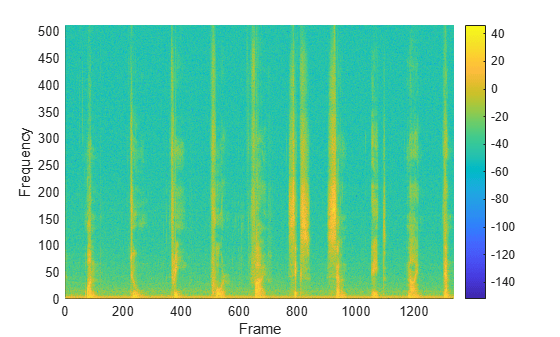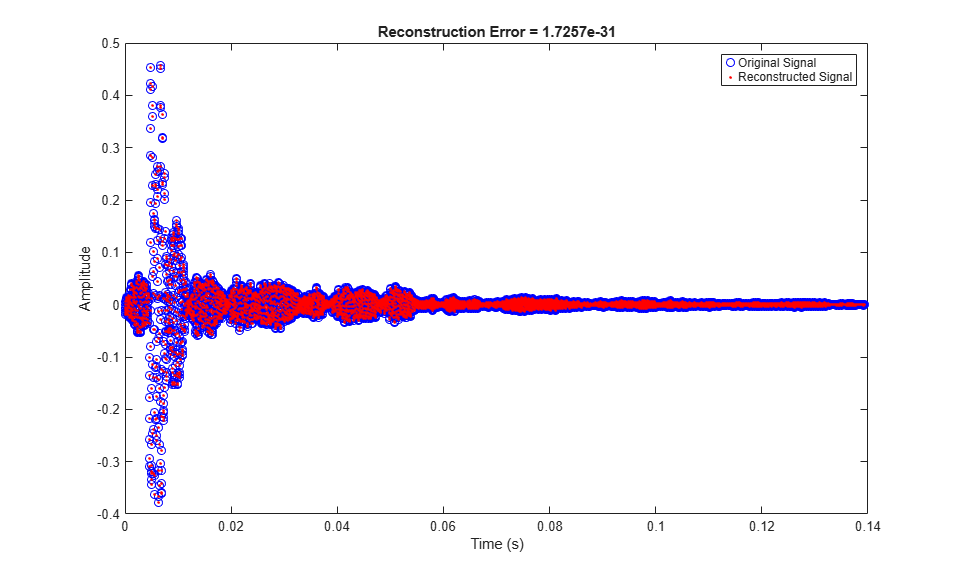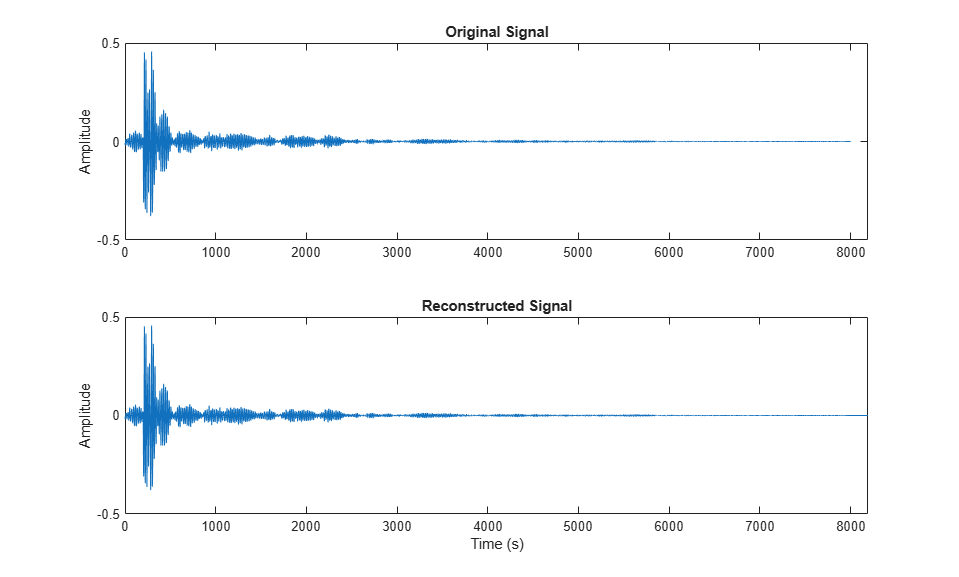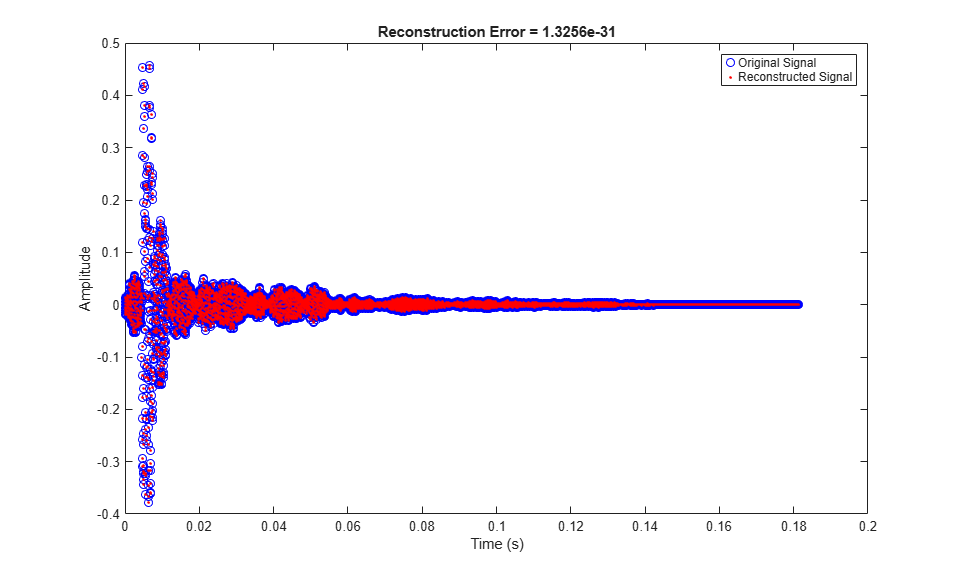# mdct

Modified discrete cosine transform

## Syntax

``Y = mdct(X,win)``
``Y = mdct(X,win,Name,Value)``
``[Y,S,Z] = mdct(___)``

## Description

example

````Y = mdct(X,win)` returns the modified discrete cosine transform (MDCT) of `X`. Before the MDCT is calculated, `X` is buffered into 50% overlapping frames that are each multiplied by the time window `win`. The function treats each column of `X` as an independent channel.```

example

````Y = mdct(X,win,Name,Value)` sets each property `Name` to the specified `Value`. Unspecified properties have default values.```
````[Y,S,Z] = mdct(___)` returns the modified discrete sine transform (MDST), `S`, and the odd discrete Fourier transform (ODFT), `Z`.```

## Examples

collapse all

Read in an audio file and then calculate the MDCT using a 1024-point Kaiser-Bessel-derived window.

```audioIn = audioread('Counting-16-44p1-mono-15secs.wav'); coef = mdct(audioIn,kbdwin(1024));```

Plot the power of the MDCT coefficients over time.

```surf(pow2db(coef.^2),'EdgeColor','none'); view([0 90]) xlabel('Frame') ylabel('Frequency') axis([0 size(coef,2) 0 size(coef,1)]) colorbar```To enable perfect reconstruction, the `mdct` function zero-pads the front and back of the audio input signal. The signal returned from `imdct` removes the zero padding added for perfect reconstruction.

Read in an audio file, create a 2048-point Kaiser-Bessel-derived window, and then clip the audio signal so that its length is a multiple of 2048.

```[x,fs] = audioread('Click-16-44p1-mono-0.2secs.wav'); win = kbdwin(2048); xClipped = x(1:end - rem(size(x,1),numel(win))); ```

Convert the signal to the frequency domain, and then reconstruct it back in the time domain. Plot the original and reconstructed signals and display the reconstruction error.

```C = mdct(xClipped,win); y = imdct(C,win); figure(1) t = (0:size(xClipped,1)-1)'/fs; plot(t,xClipped,'bo',t,y,'r.') legend('Original Signal','Reconstructed Signal') title(strcat("Reconstruction Error = ",num2str(mean((xClipped-y).^2)))) xlabel('Time (s)') ylabel('Amplitude') ```You can perform the MDCT and IMDCT without input padding using the `PadInput` name-value pair. However, there will be a reconstruction error in the first half-frame and last half-frame of the signal.

```C = mdct(xClipped,win,'PadInput',false); y = imdct(C,win,'PadInput',false); figure(2) t = (0:size(xClipped,1)-1)'/fs; plot(t,xClipped,'bo',t,y,'r.') legend('Original Signal','Reconstructed Signal') title(strcat("Reconstruction Error (Without Input Padding) = ",num2str(mean((xClipped-y).^2)))) xlabel('Time (s)') ylabel('Amplitude') ```If you specify an input signal to the `mdct` that is not a multiple of the window length, then the input signal is padded with zeros. Pass the original unclipped signal through the transform pair and compare the original signal and the reconstructed signal.

```C = mdct(x,win); y = imdct(C,win); figure(3) subplot(2,1,1) plot(x) title('Original Signal') ylabel('Amplitude') axis([0,max(size(y,1),size(x,1)),-0.5,0.5]) subplot(2,1,2) plot(y) title('Reconstructed Signal') xlabel('Time (s)') ylabel('Amplitude') axis([0,max(size(y,1),size(x,1)),-0.5,0.5]) ```The reconstructed signal is padded with zeros at the back end. Remove the zero-padding from the reconstructed signal, plot the original and reconstructed signal, and then display the reconstruction error.

```figure(4) y = y(1:size(x,1)); t = (0:size(x,1)-1)'/fs; plot(t,x,'bo',t,y,'r.') legend('Original Signal','Reconstructed Signal') title(strcat("Reconstruction Error = ",num2str(mean((x-y).^2)))) xlabel('Time (s)') ylabel('Amplitude') ```Create a `dsp.AudioFileReader` object to read in audio data frame-by-frame. Create a `dsp.SignalSink` to log the reconstructed signal for comparison. Create a `dsp.AsyncBuffer` to buffer the input stream.

```fileReader = dsp.AudioFileReader('FunkyDrums-44p1-stereo-25secs.mp3'); logger = dsp.SignalSink; buff = dsp.AsyncBuffer;```

Create a 512-point Kaiser-Bessel-derived window.

```N = 512; win = kbdwin(N);```

In an audio stream loop:

1. Read a frame of data from the file.

2. Write the frame of data to the async buffer.

3. If half a frame of data is present, read from the buffer and then perform the transform pair. Overlap-add the current output from `imdct` with the previous output, and log the results. Update the memory.

```mem = zeros(N/2,2); % initialize an empty memory while ~isDone(fileReader) audioIn = fileReader(); write(buff,audioIn); while buff.NumUnreadSamples >= N/2 x = read(buff,N,N/2); C = mdct(x,win,'PadInput',false); y = imdct(C,win,'PadInput',false); logger(y(1:N/2,:)+mem) mem = y(N/2+1:end,:); end end % Perform the transform pair one last time with a zero-padded final signal. x = read(buff,N,N/2); C = mdct(x,win,'PadInput',false); y = imdct(C,win,'PadInput',false); logger(y(1:N/2,:)+mem) reconstructedSignal = logger.Buffer;```

Read in the entire original audio signal. Trim the front and back zero padding from the reconstructed signal for comparison. Plot one channel of the original and reconstructed signals and display the reconstruction error.

```[originalSignal,fs] = audioread(fileReader.Filename); signalLength = size(originalSignal,1); reconstructedSignal = reconstructedSignal((N/2+1):(N/2+1)+signalLength-1,:); t = (0:size(originalSignal,1)-1)'/fs; plot(t,originalSignal(:,1),'bo',t,reconstructedSignal(:,1),'r.') legend('Original Signal','Reconstructed Signal') title(strcat("Reconstruction Error = ", ... num2str(mean((originalSignal-reconstructedSignal).^2,'all')))) xlabel('Time (s)') ylabel('Amplitude')```## Input Arguments

collapse all

Input array, specified as a column vector or matrix. If specified as a matrix, the columns are treated as independent audio channels.

Data Types: `single` | `double`

Window applied in the time domain, specified as an even-length vector. The transform performed by `mdct` has the same number of points as `win`. To enable perfect reconstruction, use a window that satisfies the Princen-Bradley condition (${w}_{n}^{2}+{w}_{n+N}^{2}=1$), such as a sine window or `kbdwin`.

Data Types: `single` | `double`

### Name-Value Arguments

Specify optional pairs of arguments as `Name1=Value1,...,NameN=ValueN`, where `Name` is the argument name and `Value` is the corresponding value. Name-value arguments must appear after other arguments, but the order of the pairs does not matter.

Before R2021a, use commas to separate each name and value, and enclose `Name` in quotes.

Example: `'PadInput',false`

Flag to pad input array, specified as the comma-separated pair consisting of '`PadInput`' and `true` or `false`. If set to `true`, zero-padding is added to the input `X` at both ends to enable perfect reconstruction. The number of zeros at each end is `numel(win)/2`.

Data Types: `logical`

## Output Arguments

collapse all

Modified discrete cosine transform (MDCT), returned as a vector, matrix, or 3-D array. The dimensions of `Y` are L-by-M-by-N, where:

• L –– Number of points in the frequency-domain representation of each frame, equal to `numel(win)/2`.

• M –– Number of frames the input array is partitioned into.

• If `PadInput` is set to `true`, ```M = ceil(2*size(X,1)/numel(win))+1```.

• If `PadInput` is set to `false`, ```M = ceil(2*size(X,1)/numel(win))-1```.

• N –– Number of channels, equal to `size(X,2)`.

Trailing singleton dimensions are removed from the output `Y`.

Data Types: `single` | `double`

Modified discrete sine transform (MDST), returned as a vector, matrix, or 3-D array. The dimensions of `S` are the same as the MDCT output, `Y`.

Data Types: `single` | `double`

Half-sided odd discrete Fourier transform (ODFT), returned as a vector, matrix, or 3-D array of complex numbers. The dimensions of `Z` are the same as the MDCT output, `Y`.

To construct the complete (two-sided) ODFT, mirror the half-sided ODFT: `cat(1,Z,conj(flip(Z,1)))`.

Data Types: `single` | `double`
Complex Number Support: Yes

## Algorithms

The modified discrete cosine transform is a time-frequency transform. Given an input signal `X` and window `win`, the `mdct` function performs the following steps for each independent channel:

1. The frame size is the number of elements in the specified window, N = `numel(win)`. By default, `PadInput` is set to `true`, so the input signal `X` is padded with N/2 zeros on the front and back. If the input signal is not divisible by N, additional padding is added on the back. After padding, the input signal is buffered into 50% overlapped frames.

2. Each frame of the buffered and padded input signal is multiplied by the window, `win`.

3. The input is converted into a frequency representation using the modified discrete cosine transform:

`$Y\left(k\right)=\sum _{n=0}^{N-1}X\left(n\right)\mathrm{cos}\left[\frac{\pi }{\left(N}{2}\right)}\left(n+\frac{\left(N}{2}\right)+1}{2}\right)\left(k+\frac{1}{2}\right)\right]\text{\hspace{0.17em}},\text{ }k=0,1,...,\left(N}{2}\right)-1$`

To take advantage of the FFT algorithm, the MDCT is calculated by first calculating the odd DFT:

`${Y}_{\text{O}}\left(k\right)=\sum _{n=0}^{N-1}X\left(n\right){e}^{-j\frac{\pi n}{N}\left(2k+1\right)}\text{\hspace{0.17em}},\text{ }k=0,1,...,N-1$`

and then calculating the MDCT:

`$Y\left(k\right)=\Re e\left\{{Y}_{\text{o}}\left(k\right)\right\}\mathrm{cos}\left(\frac{\pi }{N}\left(k+\frac{1}{2}\right)\left(1+\frac{N}{2}\right)\right)\text{\hspace{0.17em}},\text{ }k=0,1,...,\left(N}{2}\right)-1$`

If a second argument is requested from the `mdct` function, the modified discrete sine transform (MDST) is also computed and returned:

`$X\left(k\right)=\Im m\left\{{X}_{\text{o}}\left(k\right)\right\}\mathrm{sin}\left(\frac{\pi }{N}\left(k+\frac{1}{2}\right)\left(1+\frac{N}{2}\right)\right)\text{\hspace{0.17em}},\text{ }k=0,1,...,\left(N}{2}\right)-1$`

 Princen, J., A. Johnson, and A. Bradley. "Subband/Transform Coding Using Filter Bank Designs Based on Time Domain Aliasing Cancellation." IEEE International Conference on Acoustics, Speech, and Signal Processing (ICASSP). 1987, pp. 2161–2164.

 Princen, J., and A. Bradley. "Analysis/Synthesis Filter Bank Design Based on Time Domain Aliasing Cancellation." IEEE Transactions on Acoustics, Speech, and Signal Processing. Vol. 34, Issue 5, 1986, pp. 1153–1161.